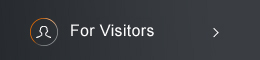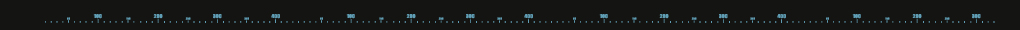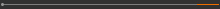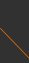﻿ nctsSponsored by[ Events ]

﻿
Activity Search
Sort out
 select all Seminars and Talks Conference and Workshop Lectures and Courses ElseField
 select all Number Theory and Representation Theory Algebraic Geometry Differential Geometry and Geometric Analysis Differential Equations and Stochastic Analysis Scientific Computing Interdisciplinary Studies Laboratory of Data Science Harmonic Analysis OthersYear
 select 2015 2016 2017 2018 2019 2020 2021 2022 2023Taiwan Mathematics School: Introduction to Riemann SurfacesEvery Wednesday, 10:20-13:10, September 15, 2021 - January 21, 2022 R509, Cosmology Building, NTU Speaker: Ching-Jui Lai (National Cheng Kung University) Organizers: Ching-Jui Lai (National Cheng Kung University) 1. Background The subject of compact Riemann surfaces or algebraic curves has its origin going back to the work of Riemann. Its development requires ideas from analysis, PDE, differential geometry, complex geometry, algebra, and topology e.t.c. This course serves as a introductory course to more general theories of complex manifold and higher dimensional algebraic geometry. 2. Outline In this course, we will introduce the notion of Riemann surfaces, holomorphic functions, meromorphic functions, differential forms on Riemann surfaces, maps between Riemann surfaces, Riemann-Roch Theorem, Abel-Jacobi theorem. We might discuss the existence of meromorphic functions and the uniformization theorem if time allows. Tentative plan: Formal definition of a Riemann surface: From CP^1 to real/complex manifolds. Examples of Riemann surfaces: quotient, graph, affine curves, projective spaces and projective curves Holomorphic and meromorphic functions Rational polynomials and theta functions Holomorphic maps of Riemann surfaces Hurwitz formula: proof and applications Differential forms, Stoke Theorem, and Residue Theorem Holomorphic one forms and Abel-Jacobi map  Theory of divisors  Finiteness theorem and Riemann-Roch formula  Applications of Riemann-Roch formula  Residue map and Serre duality    Existence of meromorphic functions (from calculus of variations, harmonic functions, to constructions of meromorphic fucntions)  A proof of Riemann-Roch formula  Uniformisaztion Theorem **2022/1/5 [ in-person class] Presentation in NCKU (國立成功大學) **2022/1/12 [ in-person class] Presentation in NCKU (國立成功大學) 3. Evaluation Attendance and Discussion 20% Homework 30% Final project 50% 4. Reference: Rick Miranda, Algebraic Curves and Riemann Surfaces, (Graduate Studies in Msrhematics, Volume 5) Simon Donaldson, Riemann Surfaces, 2011. 5. Credit: 3 Contact: murphyyu@ncts.ntu.edu.tw(C) 2021 National Center for Theoretical Sciences